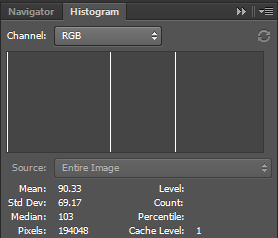# How to calculate StdDev for RGB image? [closed]

Hello,

I'm trying to get the mean and standard deviation on a RGB image. I have used the example code and I have this:

cv::Mat image = cv::imread("C:/stddev.jpg");

cv::Scalar mean, stddev; //0:1st channel, 1:2nd channel and 2:3rd channel

meanStdDev(image, mean, stddev, cv::Mat());
std::cout << "Standard deviation channel B,G,R: " << stddev << std::endl;
std::cout << "Mean B,G,R: " << mean << std::endl;


I have used this picture:And this is my output:

Standard deviation channel B,G,R: [0,0,0,0] Mean B,G,R: [1, 103, 168, 0]

In Adobe Photoshop it gives me the same values for each seperate channel but when I select RGB (So for all channels I suppose) it gives me a standard deviation of 69.17 and a mean of 90.33:So how do I get those numbers instead of each channel in OpenCV?

edit retag reopen merge delete